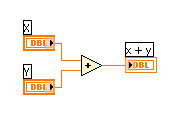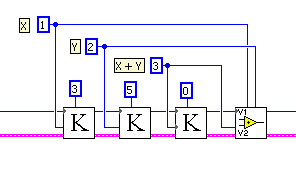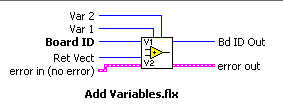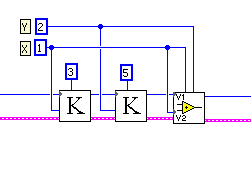# Understanding Variable Arithmetic in Onboard Programming

Publish Date: Sep 06, 2016 | 1 Ratings | 4.00 out of 5 | Print

This section contains a summary of the available math functions on general-purpose variables. Variables can be loaded, added, multiplied, AND-ed, and so on before being used as data in a motion control function. General-purpose variables are 32 bits long and can be used either signed (i32) or unsigned (u32). All functions in this section operate on 32-bit values and return 32-bit values. You must be careful to avoid overflow and underflow conditions. For example, multiplying two 32-bit variables and returning the result to a 32-bit variable might overflow and wrap around. Smaller sized data is right aligned within a 32-bit variable. Bit wise logical functions always assume this alignment and return similarly aligned results. Many Flexmotion functions can take input data from a general-purpose variable by pointing to the variable with the input vector parameter. Similarly, all read functions can return data to a general-purpose variable by using the return vector parameter. See the next section ‘Understanding Input and Return Vectors in Onboard Programming’ for a detailed description of input and return vectors. All data operation functions set condition codes (less than, equal to or greater than zero) depending on the result of the operation. Your program can test these conditions with the Jump to Label on Condition function. Executing a data operation function with a return vector of zero (0) tells the program to set the condition code and then throw the resulting data away. In this way, you can use all the data operations functions as tests for conditional branching. You can use indirect variables as variable inputs or return vectors with all data operations functions. Let’s show an example in LabVIEW:

Table 1.In this code we have two variables ‘x’ and ‘y’ and we are adding them, the result here is stored in a third variable. In C you will have the following: z = x + y; Or this if you want to same variable to keep the new value: x = x + y;

In onboard programming you would use the data operation functions:Figure 1.Figure 2.

Wiring diagram of the Add Variables function. We are declaring three variables, 0x01, 0x02 and 0x03, with values 3, 5 and 0 respectively, then we add variable 0x01 and 0x02 and we specify that the ‘return vector’ or place to store the result will be in 0x03. So after executing this code you will have ‘8’ in 0x03. If we would like to save space and want to store the result in one of the variables been added you just wire the same variable to the return vector, like this:Figure 3.

Which is equivalent to: x = x + y in C. In the arithmetic functions you have access to the following operations:

1. Add
2. Subtract
3. Multiply
4. Divide
5. AND
6. OR
7. Ex-OR
8. Invert (NOT)
9. Logical Shift
10. Load Constant to a variable
11. Read Constant from a variable

If you would like to have specific information on how to use each of the functions please check the LabVIEW Online Help for each individual function or best of all consult the ‘Flexmotion Software Reference Manual’ for more information. Some of the help documents have examples to demonstrate its use.

For all number functions (add, subtract, multiply, divide) the result can be returned to a new variable or one of the two input variables, returned to the host computer, or thrown away. In all cases the EQUAL condition code is set if the result is equal to zero (0) and GREATER THAN and LESS THAN in case the value is greater than zero or less than zero respectively.

For all bit functions, the result can be returned to a new variable or one of the two input variables, returned to the host computer, or thrown away. In all cases the EQUAL condition code is set True if the result equals zero (all bits low) and False if any bit is set. The GREATER THAN and LESS THAN codes are also set but can be confusing after logical bit wise operations. For the bit functions examples are as follows:

AND.
If the values in Var 1 and Var 2 are 0x0000 1234 and 0x0000 EEEE, respectively, the result of the bit wise AND is 0x0000 0224, which is NOT EQUAL to zero.

OR.
If the values in Var 1 and Var 2 are 0x5A5A 1234 and 0x8282 0000, respectively, the result of the bit wise OR is 0xDADA 1234, which is NOT EQUAL to zero.

Ex-OR.
If the values in Var 1 and Var 2 are 0x5A5A 1234 and 0xFFFF 4321, respectively, the result of the bit wise XOR is 0xA5A5 5115, which is NOT EQUAL to zero.

Invert.
If the value in Var 1 is 0x0000 5A5A, the result of the bit wise NOT is 0xFFFF A5A5. The EQUAL condition code is set to False.

Logical Shift.
If the value in Var 1 is 0x0000 F002 and Logical Shift = –1, this VI returns 0x00007801.

With all this functionality you can program complex arithmetic operations inside an onboard program, which gives you more options to migrate your code to the onboard processor. Is always a good practice to read thoroughly the software manual so you will be aware of ‘all’ the important notes and features of a function.

### Ratings

Rate this document

Answered Your Question?
Yes No